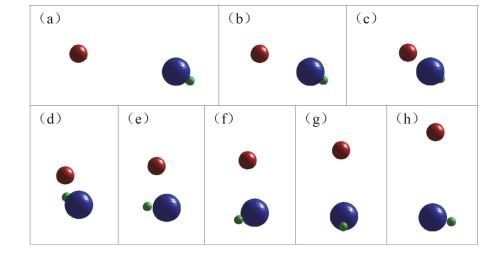## Design for Visualized Presentation of Microcosmic Chemical Reaction Process Based on Matlab GUI

Xia Yue, Yang Cong, Duan Zhixin,Abstract

Based on the quasi-classical trajectory (QCT) method and Matlab GUI technology, we developed a program code for visualizing the collision process of the elementary chemical reactions of the a + bc type. The general methodology of QCT, abstraction of dynamical properties of molecular collisions and the making of Graphical User Interface are introduced. The running results of an application to the reaction F + HCl→HF + Cl is also presented. The results showed that this program could vividly demonstrate the behavior and final state of the atom-diatom collision process in animated form. Students can interact with internal MATLAB code through graphical user interface, observe the reactive behavior and final results in real-time from multiple angles, which helps students to understand the complex reaction mechanism and deepen their perceptual impression of the chemical process at a microscopic atomic/molecular level.

Keywords： Molecular reaction dynamics ; Chemical reaction ; Visualization ; Quasi-classical trajectory method ; Matlab GUI

Xia Yue. Design for Visualized Presentation of Microcosmic Chemical Reaction Process Based on Matlab GUI. University Chemistry[J], 2020, 35(9): 185-193 doi:10.3866/PKU.DXHX201908046

## 2 准经典轨线方法

$B = \left[ {\begin{array}{*{20}{c}} 0 \\ 0 \\ 0 \end{array}} \right], \begin{array}{*{20}{c}} {}&{} \end{array}C = \left[ {\begin{array}{*{20}{c}} {{Q_1}} \\ {{Q_2}} \\ {{Q_3}} \end{array}} \right], \begin{array}{*{20}{c}} {}&{} \end{array}A = \left[ {\begin{array}{*{20}{c}} {{Q_4}} \\ {{Q_5}} \\ {{Q_6}} \end{array}} \right] + \frac{{{M_{\rm{a}}}}}{{{M_{\rm{b}}} + {M_{\rm{c}}}}}\left[ {\begin{array}{*{20}{c}} {{Q_1}} \\ {{Q_2}} \\ {{Q_3}} \end{array}} \right]$

${\rm{O}} = \frac{{{M_{\rm{a}}}A + {M_{\rm{b}}}B + {M_{\rm{c}}}C}}{{{M_{\rm{a}}} + {M_{\rm{b}}} + {M_{\rm{c}}}}} = \frac{1}{{{M_{\rm{a}}} + {M_{\rm{b}}} + {M_{\rm{c}}}}}\left[ {\begin{array}{*{20}{c}} {{M_{\rm{c}}}{Q_1} + {M_{\rm{a}}}{Q_4} + \frac{{{M_{\rm{a}}}{M_{\rm{c}}}}}{{{M_{\rm{b}}} + {M_{\rm{c}}}}}{Q_1}} \\ {{M_{\rm{c}}}{Q_2} + {M_{\rm{a}}}{Q_5} + \frac{{{M_{\rm{a}}}{M_{\rm{c}}}}}{{{M_{\rm{b}}} + {M_{\rm{c}}}}}{Q_2}} \\ {{M_{\rm{c}}}{Q_3} + {M_{\rm{a}}}{Q_6} + \frac{{{M_{\rm{a}}}{M_{\rm{c}}}}}{{{M_{\rm{b}}} + {M_{\rm{c}}}}}{Q_3}} \end{array}} \right]$

$OA = A - O;OB = A - B;OC = C - O$

### 3.1 动画主界面设计

Matlab的GUI功能可以开发出功能友好的图形界面，利用图形用户界面开发环境GUIDE可以通过简单的鼠标拖曳等操作设计出自己的GUI程序界面图1显示了微观化学反应动画主界面的整体布局。主界面包含三个主要部分：左上侧的“选择数据”部分，左下侧“控制按钮”部分，以及右侧的“动画演示”部分。弹出式菜单“选择文件”，用于选择Fortran程序包计算得到的数据文件。a、b、c三个原子的质量和半径可以在主界面的6个编辑框内直接输入，也可以在M格式的文件中进行修改(修改后点击“刷新”按钮即可显示数据)。为了更好地观察动画演示过程，我们还设置了碰撞原子的颜色选择按钮、时间步数编辑框和观察视角选择框。时间步数默认20个时间步显示一帧动画，当碰撞过程进行比较快时，可以调节时间步的大小；观察视角框提供了6个不同的方位视角对碰撞过程进行观察。在左下侧，四个控制按钮“Play、Pause、Continue、Stop”可以对运行中的动画进行控制。

### 图1### 3.2 回调函数的编写

(1) Fortran计算结果文件的读取

function pb1_Callback(hObject, eventdata, handles)

global Y;

[yDate, yPath]=uigetfile({'*.*'});

yPath2 = strcat(yPath, yDate);

set(handles.tx1, 'string', yPath2);

(2)原子质量、半径、颜色的选择

function pushbutton12_Callback(hObject, eventdata, handles)

global Ma Mb Mc;

global Na Nb Nc;

global Nstep;

set(handles.edit1, 'string', Ma);

set(handles.edit2, 'string', Mb);

set(handles.edit3, 'string', Mc);

set(handles.edit4, 'string', Na);

set(handles.edit5, 'string', Nb);

set(handles.edit6, 'string', Nc);

set(handles.edit7, 'string', Nstep);

function edit1_Callback(hObject, eventdata, handles)

global Ma;

Ma=str2num(get(hObject, 'string'));

function edit1_CreateFcn(hObject, eventdata, handles)

global Ma;

set(hObject, 'string', Ma);

end

(3)坐标变换、球体设置以及动画运行：

B=;

C=Y(:, 1:3);

M1=Mc/(Mb+Mc);

Ms=Ma+Mb+Mc;

for i=1:3

A(:, i)=M1*Y(:, i)+Y(:, i+3);

end

O=(Ma*A+Mb*B+Mc*C)/Ms;

Oa=A-O;

Ob=B-O;

Oc=C-O;     %坐标变换结束

[xa, ya, za]=sphere(30); % A原子的球体设置

xa=Na*xa;

ya=Na*ya;

za=Na*za;

B、C原子球体表示省略；

for i=1:3

T(:, i)=Y(:, 13); %时间提取

end

yPau=1;

ySt=1;     %动画演示控制变量

videoif=0;

videoif=get(handles.videout, 'value');

Nmax=length(oa);

VideoY=moviein(floor(Nmax/Nstep));

axes(handles.axes1);

videoi=0;

for i=1:Nstep:Nmax

videoi=videoi+1;

if ySt==0

break;

end

if yPau==0

while yPau==0

pause(0.2);

view(yView);

end

end

surf(za+oa(i, 3), xa+oa(i, 1), ya+oa(i, 2), 'EdgeColor', 'none', 'FaceColor', yACo);

hold on;

surf(zb+ob(i, 3), xb+ob(i, 1), yb+ob(i, 2), 'EdgeColor', 'none', 'FaceColor', yBCo);

surf(zc+oc(i, 3), xc+oc(i, 1), yc+oc(i, 2), 'EdgeColor', 'none', 'FaceColor', yCCo);

axis equal

light

axis([-8, 15, -5, 5, -5, 5]);

view(yView);     %设置yview数值可以从不同视角演示动画

axis off

title(T(i, 1));

if(videoif==1)

VideoY(:, videoi)=getframe;

end

hold off

pause(0.001)

end

fps=str2num(get(handles.fps, 'string'));

picqu=str2num(get(handles.picqu, 'string'));

movie(VideoY, 1);

if(videoif==1)

movie2avi(VideoY, 'out.avi', 'fps', fps, 'quality', picqu);

end

(4)动画运行的控制

Ⅰ.动画展示步数的选择，按积分时间步的倍数进行动画展示，步数越小，动画帧数越多。

function edit7_CreateFcn(hObject, eventdata, handles)

global Nstep;

set(hObject, 'string', Nstep);

end

Ⅱ.动画的运行、暂停、复位、停止利用两个时间参数来控制。yst为全局控制变量，yst = 1时，动画开始运行，yst = 0时动画stop；ypau为循环内变量，ypau = 0时动画暂停，ypau = 1时继续运行。

### 4.2 动画演示并输出视频文件

 a + bc F + HCl 相对质量/amu 原子半径/nm a原子 F 19 6.4 b原子 H 1.008 3.2 c原子 Cl 35.45 9.9

### 图2#### 4.3.1 碰撞类型

F + HCl碰撞可以生成新的产物分子HF，发生化学反应；也可以通过碰撞将能量传递给反应物分子HCl，改变HCl分子的振动、转动状态，但不生成新的分子；在碰撞能较高时(如1254.6 kJ·mol−1)，还可以将HCl分子解离成原子状态。这三种不同的碰撞过程分别如图345所示。

### 图3### 图4### 图5### 图6## 参考文献 原文顺序 文献年度倒序 文中引用次数倒序 被引期刊影响因子

Camargo P. H. C. J. Mater. Sci. 2019, 54 (15), 10595.

Blais N. C. ; Truhlar D. G. ; Garrett B. C. J. Chem. Phys. 1983, 78 (5), 2363.

Hase W. L. ; Duchovic R. J. ; Hu X. ; Komornicki A. ; Lim K. ; Lu D. H. ; Peslherbe G. H. ; Swamy K. N. ; Vande L. S. R. ; Varandas A. ; et al QCPE Bull. 1996, 16 (4), 43.

Duan Z. X. ; Li W. L. ; Qiu M. H. J. Chem. Phys. 2012, 136, 2244303.

/

 〈〉